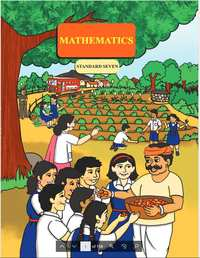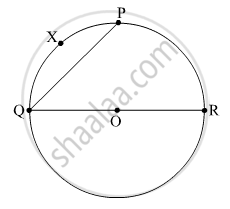# Balbharati solutions for Mathematics 7th Standard Maharashtra State Board chapter 11 - Circle [Latest edition]

#### Chapters## Chapter 11: Circle

Practice Set 42Practice Set 43
Practice Set 42 [Page 77]

### Balbharati solutions for Mathematics 7th Standard Maharashtra State Board Chapter 11 Circle Practice Set 42 [Page 77]

Practice Set 42 | Q 1.1 | Page 77

Complete the table below.

 Radius (r) Diameter (d) Circumference (c) 7 cm ______ _______
Practice Set 42 | Q 1.2 | Page 77

Complete the table below.

 Radius (r) Diameter (d) Circumference (c) _______ 28 cm _________
Practice Set 42 | Q 1.3 | Page 77

Complete the table below.

 Radius (r) Diameter (d) Circumference (c) _______ _________ 616 cm
Practice Set 42 | Q 1.4 | Page 77

Complete the table below.

 Radius (r) Diameter (d) Circumference (c) _______ _________ 72.6 cm
Practice Set 42 | Q 2 | Page 77

If the circumference of a circle is 176 cm, find its radius.

Practice Set 42 | Q 3 | Page 77

The radius of a circular garden is 56 m. What would it cost to put a 4-round fence around this garden at a rate of 40 rupees per metre?

Practice Set 42 | Q 4 | Page 77

The wheel of a bullock cart has a diameter of 1.4 m. How many rotations will the wheel complete as the cart travels 1.1 km?

Practice Set 43 [Page 79]

### Balbharati solutions for Mathematics 7th Standard Maharashtra State Board Chapter 11 Circle Practice Set 43 [Page 79]

Practice Set 43 | Q 1 | Page 79
Choose the correct option.
If arc AXB and arc AYB are corresponding arcs and m(arc AXB) = 120° then m(arc AYB) = _______
• 140°

• 60°

• 240°

• 160°

Practice Set 43 | Q 2 | Page 79

Some arcs are shown in the circle with centre ‘O’. Write the names of the minor arcs, major arcs, and semicircular arcs from among them.Practice Set 43 | Q 3 | Page 79

In a circle with centre O, the measure of a minor arc is 110°. What is the measure of the major arc PYQ?

## Chapter 11: Circle

Practice Set 42Practice Set 43## Balbharati solutions for Mathematics 7th Standard Maharashtra State Board chapter 11 - Circle

Balbharati solutions for Mathematics 7th Standard Maharashtra State Board chapter 11 (Circle) include all questions with solution and detail explanation. This will clear students doubts about any question and improve application skills while preparing for board exams. The detailed, step-by-step solutions will help you understand the concepts better and clear your confusions, if any. Shaalaa.com has the Maharashtra State Board Mathematics 7th Standard Maharashtra State Board solutions in a manner that help students grasp basic concepts better and faster.

Further, we at Shaalaa.com provide such solutions so that students can prepare for written exams. Balbharati textbook solutions can be a core help for self-study and acts as a perfect self-help guidance for students.

Concepts covered in Mathematics 7th Standard Maharashtra State Board chapter 11 Circle are Arc of the Circle, Circumference of a Circle, Concept of Circle - Centre, Radius, Diameter, Arc, Sector, Chord, Segment, Semicircle, Circumference, Interior and Exterior, Concentric Circles, Central Angle and the Measure of an Arc.

Using Balbharati 7th Standard solutions Circle exercise by students are an easy way to prepare for the exams, as they involve solutions arranged chapter-wise also page wise. The questions involved in Balbharati Solutions are important questions that can be asked in the final exam. Maximum students of Maharashtra State Board 7th Standard prefer Balbharati Textbook Solutions to score more in exam.

Get the free view of chapter 11 Circle 7th Standard extra questions for Mathematics 7th Standard Maharashtra State Board and can use Shaalaa.com to keep it handy for your exam preparation